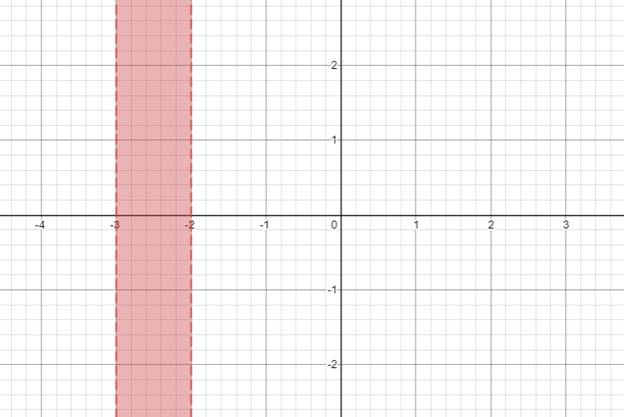# The solution of given nonlinear inequality equation and plot a graph for it.### Precalculus: Mathematics for Calcu...

6th Edition
Stewart + 5 others
Publisher: Cengage Learning
ISBN: 9780840068071### Precalculus: Mathematics for Calcu...

6th Edition
Stewart + 5 others
Publisher: Cengage Learning
ISBN: 9780840068071

#### Solutions

Chapter 1.7, Problem 40E
To determine

## The solution of given nonlinear inequality equation and plot a graph for it.

Expert Solution

3<x<2

### Explanation of Solution

Given:

Non-linear inequality equation

x2+5x+6>0

Calculation,

Non-linear inequality equation,

x2+5x+6>0x2+(3+2)x+6>0x2+3x+2x+6>0x(x+3)+2(x+3)>0(x+3)(x+2)>0Now,(x+3)>0x>3and,(x+2)<0x<2so,3<x<2

Now, graph of x2+5x+6>0Conclusion:

Hence, the values of x lies between the -3 and -2.

### Have a homework question?

Subscribe to bartleby learn! Ask subject matter experts 30 homework questions each month. Plus, you’ll have access to millions of step-by-step textbook answers!